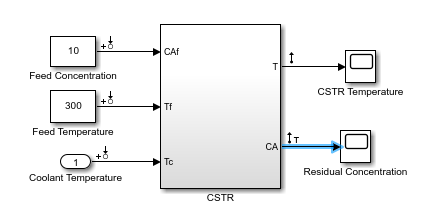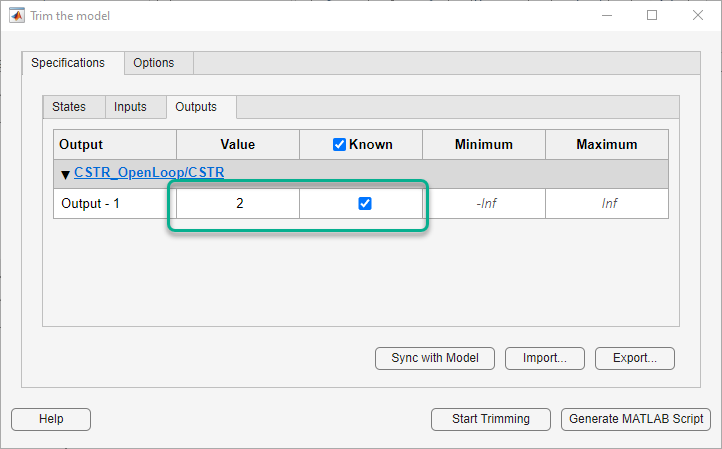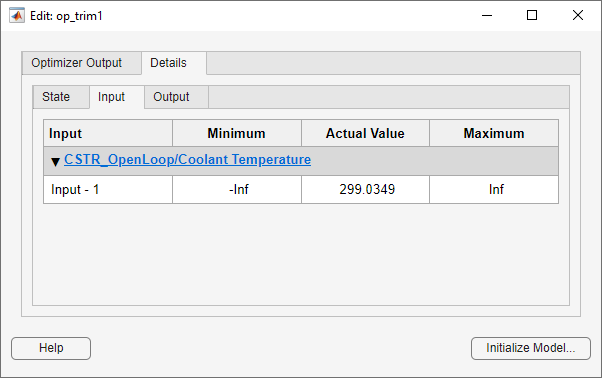Generally, real systems are nonlinear. To design an MPC controller for a nonlinear system, you can model the plant in Simulink®.

Although an MPC controller can regulate a nonlinear plant, the model used within the controller must be linear. In other words, the controller employs a linear approximation of the nonlinear plant. The accuracy of this approximation significantly affects controller performance.

To obtain such a linear approximation, you linearize the nonlinear plant at a specified operating point.

Note

Simulink Control Design™ software must be installed to linearize nonlinear Simulink models.

You can linearize a Simulink model:

### Linearization Using MATLAB Code

This example shows how to obtain a linear model of a plant using a MATLAB script.

For this example the CSTR model, `CSTR_OpenLoop`, is linearized. The model inputs are the coolant temperature (manipulated variable of the MPC controller), limiting reactant concentration in the feed stream, and feed temperature. The model states are the temperature and concentration of the limiting reactant in the product stream. Both states are measured and used for feedback control.

The operating point defines the nominal conditions at which you linearize a model. It is usually a steady-state condition.

Suppose that you plan to operate the CSTR with the output concentration, `C_A`, at $2\phantom{\rule{0.2777777777777778em}{0ex}}kmol/{m}^{3}$. The nominal feed concentration is $10\phantom{\rule{0.2777777777777778em}{0ex}}kmol/{m}^{3}$, and the nominal feed temperature is 300 K. Create an operating point specification object to define the steady-state conditions.

```opspec = operspec('CSTR_OpenLoop'); opspec = addoutputspec(opspec,'CSTR_OpenLoop/CSTR',2); opspec.Outputs(1).Known = true; opspec.Outputs(1).y = 2; op1 = findop('CSTR_OpenLoop',opspec);```
``` Operating point search report: --------------------------------- Operating point search report for the Model CSTR_OpenLoop. (Time-Varying Components Evaluated at time t=0) Operating point specifications were successfully met. States: ---------- (1.) CSTR_OpenLoop/CSTR/C_A x: 2 dx: -4.6e-12 (0) (2.) CSTR_OpenLoop/CSTR/T_K x: 373 dx: 5.48e-11 (0) Inputs: ---------- (1.) CSTR_OpenLoop/Coolant Temperature u: 299 [-Inf Inf] Outputs: ---------- (1.) CSTR_OpenLoop/CSTR y: 2 (2) ```

The calculated operating point is `C_A` = $2\phantom{\rule{0.2777777777777778em}{0ex}}kmol/{m}^{3}$ and `T_K` = 373 K. Notice that the steady-state coolant temperature is also given as 299 K, which is the nominal value of the manipulated variable of the MPC controller.

To specify:

• Values of known inputs, use the `Input.Known` and `Input.u` fields of `opspec`

• Initial guesses for state values, use the `State.x` field of `opspec`

For example, the following code specifies the coolant temperature as 305 K and initial guess values of the `C_A` and `T_K` states before calculating the steady-state operating point:

```opspec = operspec('CSTR_OpenLoop'); opspec.States(1).x = 1; opspec.States(2).x = 400; opspec.Inputs(1).Known = true; opspec.Inputs(1).u = 305; op2 = findop('CSTR_OpenLoop',opspec);```
``` Operating point search report: --------------------------------- Operating point search report for the Model CSTR_OpenLoop. (Time-Varying Components Evaluated at time t=0) Operating point specifications were successfully met. States: ---------- (1.) CSTR_OpenLoop/CSTR/C_A x: 1.78 dx: -4.8e-14 (0) (2.) CSTR_OpenLoop/CSTR/T_K x: 377 dx: 5.4e-13 (0) Inputs: ---------- (1.) CSTR_OpenLoop/Coolant Temperature u: 305 Outputs: None ---------- ```

Specify Linearization Inputs and Outputs

If the linearization input and output signals are already defined in the model, as in `CSTR_OpenLoop`, then use the following to obtain the signal set.

`io = getlinio('CSTR_OpenLoop');`

Otherwise, specify the input and output signals as shown here.

```io(1) = linio('CSTR_OpenLoop/Coolant Temperature',1,'input'); io(2) = linio('CSTR_OpenLoop/Feed Concentration',1,'input'); io(3) = linio('CSTR_OpenLoop/Feed Temperature',1,'input'); io(4) = linio('CSTR_OpenLoop/CSTR',1,'output'); io(5) = linio('CSTR_OpenLoop/CSTR',2,'output');```

Linearize Model

Linearize the model using the specified operating point, `op1`, and input/output signals, `io`.

`sys = linearize('CSTR_OpenLoop',op1,io)`
```sys = A = C_A T_K C_A -5 -0.3427 T_K 47.68 2.785 B = Coolant Temp Feed Concent Feed Tempera C_A 0 1 0 T_K 0.3 0 1 C = C_A T_K CSTR/1 0 1 CSTR/2 1 0 D = Coolant Temp Feed Concent Feed Tempera CSTR/1 0 0 0 CSTR/2 0 0 0 Continuous-time state-space model. ```

### Linearization Using Model Linearizer in Simulink Control Design

This example shows how to linearize a Simulink model using the Model Linearizer, provided by the Simulink Control Design software.

This example uses the CSTR model, `CSTR_OpenLoop`.

`open_system('CSTR_OpenLoop')`

Specify Linearization Inputs and Outputs

The linearization inputs and outputs are already specified for `CSTR_OpenLoop`. The input signals correspond to the outputs from the `Feed Concentration`, `Feed Temperature`, and `Coolant Temperature` blocks. The output signals are the inputs to the `CSTR Temperature` and `Residual Concentration` blocks.

To specify a signal as a linearization input or output, first open the Linearization tab. To do so, in the Simulink Apps gallery, click Linearization Manager. Then, in the Simulink model window, click the signal.

To specify the signal as a:

• Linearization input, on the Linearization tab, in the Insert Analysis Points gallery, click Input Perturbation.

• Linearization output, on the Linearization tab, in the Insert Analysis Points gallery, click Output Measurement.

Open Model Linearizer

To open the Model Linearizer, in the gallery, click Model Linearizer.Specify Residual Concentration as Known Trim Constraint

In the Simulink model window, click the `CA` output signal from the `CSTR` block. Then, on the Linearization tab, in the Insert Analysis Points gallery, click Trim Output Constraint.In the Model Linearizer, on the Linear Analysis tab, select Operating Point > Trim Model.

In the Trim the model dialog box, on the Outputs tab:

• Select the Known check box for ```Channel - 1``` under CSTR_OpenLoop/CSTR.

• Set the corresponding Value to `2` kmol/m3.Create and Verify Operating Point

In the Trim the model dialog box, click .

The operating point `op_trim1` displays in the Linear Analysis Workspace.Double click `op_trim1` to view the resulting operating point.

In the Edit dialog box, select the Input tab.The coolant temperature at steady state is 299 K, as desired.

Linearize Model

On the Linear Analysis tab, in the Operating Point drop-down list, select `op_trim1`.

Clickto linearize the model.

This option creates the linear model `linsys1` in the Linear Analysis Workspace and generates a step response for this model. `linsys1` uses `optrim1` as its operating point.The step response from feed concentration to output `CSTR/2` displays an interesting inverse response. An examination of the linear model shows that `CSTR/2` is the residual CSTR concentration, `C_A`. When the feed concentration increases, `C_A` increases initially because more reactant is entering, which increases the reaction rate. This rate increase results in a higher reactor temperature (output `CSTR/1`), which further increases the reaction rate and `C_A` decreases dramatically.

Export Linearization Result

If necessary, you can repeat any of these steps to improve your model performance. Once you are satisfied with your linearization result, in the Model Linearizer, drag and drop it from the Linear Analysis Workspace to the MATLAB Workspace. You can now use your linear model to design an MPC controller.